-->

# Ultimate C Programming Cheat Sheet

### C Programming Cheat Sheet

What is C Program?

C  is a general-purpose, imperative computer programming language, supporting structured programming, lexical variable scope and recursion, while a static type system prevents many unintended operations. By design, C provides constructs that map efficiently to typical machine instructions, and therefore it has found lasting use in applications that had formerly been coded in assembly language, including operating systems, as well as various application software for computers ranging from supercomputers to embedded systems.

header file is a file with extension .h which contains C function declarations and macro definitions to be shared between several source files. There are two types of header files: the files that the programmer writes and the files that comes with your compiler.
2.Variables
 Declaring int x; A variable. char x = ‘A’; A variable & initializing it. float a,b,c; Multiple variables of the same type. const int n = 18; A constant variable: can’t assign to after declaration.

## 3.Integer Types.

TypeStorage sizeValue range
char1 byte-128 to 127 or 0 to 255
unsigned char1 byte0 to 255
signed char1 byte-128 to 127
int2 or 4 bytes-328 to 327 or -2,1448 to 2,147,483,647
unsigned int2 or 4 bytes0 to 65,535 or 0 to 4,294,967,295
short2 bytes-32,768 to 32,767
unsigned short2 bytes0 to 65,535
long4 bytes-2,147,483,648 to 2,147,483,647
unsigned long4 bytes0 to 4,294,967,295

### 4.Operators in C.

 Arithmetic Operators Relational Operators. Logical Operators. Bit-wise Operators. Assignment Operators. Misc Operators.

## I.Arithmetic Operators

OperatorDescriptionExample
+Adds two operands.X + Y = 20
Subtracts second operand from the first.X – Y = -20
*Multiplies both operands.X*Y = 100
/Divides numerator by de-numerator.Y / X= 1
%Modulus Operator and remainder of after an integer division.Y % X= 0
++Increment operator increases the integer value by one.X++ = 11
Decrement operator decreases the integer value by one.X– = 9

## II.Relational Operators

OperatorDescriptionExample
==Checks if the values of two operands are equal or not. If yes, then the condition becomes true.(X == Y) is not true.
!=Checks if the values of two operands are equal or not. If the values are not equal, then the condition becomes true.(X != Y) is true.
>Checks if the value of left operand is greater than the value of right operand. If yes, then the condition becomes true.(X > Y) is not true.
<Checks if the value of left operand is less than the value of right operand. If yes, then the condition becomes true.(X < Y) is true.
>=Checks if the value of left operand is greater than or equal to the value of right operand. If yes, then the condition becomes true.(X >= Y) is not true.
<=Checks if the value of left operand is less than or equal to the value of right operand. If yes, then the condition becomes true.(X <= Y) is true

## III.Logical Operators

OperatorDescriptionExample
&&Called Logical AND operator. If both the operands are non-zero, then the condition becomes true.(X && Y) is false.
||Called Logical OR Operator. If any of the two operands is non-zero, then the condition becomes true.(X || Y) is true.
!Called Logical NOT Operator. It is used to reverse the logical state of its operand. If a condition is true, then Logical NOT operator will make it false.!(X && Y) is true.

## IV. Bit-wise Operators

OperatorDescriptionExample
&Binary AND Operator copies a bit to the result if it exists in both operands.(X &Y) = 12
|Binary OR Operator copies a bit if it exists in either operand.(X | Y) = 61
^Binary XOR Operator copies the bit if it is set in one operand but not both.(X ^ Y) = 49
~Binary Ones Complement Operator is unary and has the effect of ‘flipping’ bits.(~X ) = -211,
<<Binary Left Shift Operator. The left operands value is moved left by the number of bits specified by the right operand.X << 2 = 200
>>Binary Right Shift Operator. The left operands value is moved right by the number of bits specified by the right operand.X >> 2 = 19

## V.Assignment Operators

OperatorDescriptionExample
=Simple assignment operator. Assigns values from right side operands to left side operandZ = X + Y will assign the value of X + Y to Z
+=Add AND assignment operator. It adds the right operand to the left operand and assign the result to the left operand.Z += X is equivalent to Z = Z + X
-=Subtract AND assignment operator. It subtracts the right operand from the left operand and assigns the result to the left operand.Z -= X is equivalent to Z = Z – X
*=Multiply AND assignment operator. It multiplies the right operand with the left operand and assigns the result to the left operand.Z *= X is equivalent to Z = Z * X
/=Divide AND assignment operator. It divides the left operand with the right operand and assigns the result to the left operand.Z /= X is equivalent to Z = Z/ X
%=Modulus AND assignment operator. It takes modulus using two operands and assigns the result to the left operand.Z %= X is equivalent to Z = Z % X
<<=Left shift AND assignment operator.Z <<= 2 is same as Z = Z << 2
>>=Right shift AND assignment operator.Z >>= 2 is same as Z = Z >> 2
&=Bitwise AND assignment operator.Z &= 2 is same as Z = Z & 2
^=Bitwise exclusive OR and assignment operator.Z ^= 2 is same as Z = Z ^ 2
|=Bitwise inclusive OR and assignment operator.Z |= 2 is same as Z = Z | 2

## VI.Misc Operators

OperatorDescriptionExample
sizeof()Returns the size of a variable.sizeof(x), where a is integer, will return 4.
&Returns the address of a variable.&X; returns the actual address of the variable.
*Pointer to a variable.*X;
? :Conditional Expression.If Condition is true ? then value X : otherwise value Y

# 5.Decision Making

S.N.Statement & Description
1if statementAn if statement consists of a Boolean expression followed by one or more statements.
2if…else statementAn if statement can be followed by an optional else statement, which executes when the Boolean expression is false.
3nested if statementsYou can use one if or else if statement inside another if or else if statement(s).
4switch statementA switch statement allows a variable to be tested for equality against a list of values.
5nested switch statementsYou can use one switch statement inside another switch statement(s).

# 6.Loops.

S.N.Loop Type & Description
1while loopRepeats a statement or group of statements while a given condition is true. It tests the condition before executing the loop body.
2for loopExecutes a sequence of statements multiple times and abbreviates the code that manages the loop variable.
3do…while loopIt is more like a while statement, except that it tests the condition at the end of the loop body.
4nested loopsYou can use one or more loops inside any other while, for, or do..while loop.

# 7.Functions.

## What are Functions?

function is a group of statements that together perform a task. Every C program has at least onefunction, which is main(), and all the most trivial programs can define additional functions. You can divide up your code into separate functions.

## Defining a Function

 Return Type − A function may return a value. The return_type is the data type of the value the function returns. Some functions perform the desired operations without returning a value. In this case, the return_type is the keyword void. Function Name − This is the actual name of the function. The function name and the parameter list together constitute the function signature. Function Body − The function body contains a collection of statements that define what the function does. Parameters − A parameter is like a placeholder. When a function is invoked, you pass a value to the parameter. This value is referred to as actual parameter or argument.

## Calling a Function

 #include #includeint sum(int,int);void main() { int a,b,c; printf(“\nEnter the two numbers : “); scanf(“%d%d”,&a,&b);c = sum(a,b); printf(“\nAddition of two number is : %d”,c); getch(); } int sum (int num1,int num2) { int num3; num3 = num1 + num2 ; return(num3); }

OUTPUT:
 Enter the two numbers : 12 12 Addition of two number is : 24

# 8.Arrays.

## What are Arrays?

An array is a collection of data items, all of the same type, accessed using a common name. A one-dimensional array is like a list; A two dimensionalarray is like a table; The C language places no limits on the number of dimensions in an array, though specific implementations may.
S.N.Concept & Description
1Multi-dimensional arraysC supports multidimensional arrays. The simplest form of the multidimensional array is the two-dimensional array.
2Passing arrays to functionsYou can pass to the function a pointer to an array by specifying the array’s name without an index.
3Return array from a functionC allows a function to return an array.
4Pointer to an arrayYou can generate a pointer to the first element of an array by simply specifying the array name, without any index.

# 9.Pointers.

## What are Pointers?

pointer is a variable whose value is the address of another variable, i.e., direct address of the memory location.
 int *ip; /* pointer to an integer */ double *dp; /* pointer to a double */ float *fp; /* pointer to a float */ char *ch /* pointer to a character */

S.N.Concept & Description
1Pointer arithmeticThere are four arithmetic operators that can be used in pointers: ++, –, +, –
2Array of pointersYou can define arrays to hold a number of pointers.
3Pointer to pointerC allows you to have pointer on a pointer and so on.
4Passing pointers to functions in CPassing an argument by reference or by address enable the passed argument to be changed in the calling function by the called function.
5Return pointer from functions in CC allows a function to return a pointer to the local variable, static variable, and dynamically allocated memory as well.

## NULL Pointers

It is always a good practice to assign a NULL value to a pointer variable in case you do not have an exact address to be assigned. This is done at the time of variable declaration. A pointer that is assigned NULL is called a null pointer.
 #include int main () { int *ptr = NULL; printf(“The value of ptr is : %x\n”, ptr ); return 0; }

OUTPUT:
 The value of ptr is 0

# 10.Strings.

What are Strings?
string in C is merely an array of characters. The length of a string is determined by a terminating null character: ‘\0’ . So, a string with the contents, say, “abc” has four characters: ‘a’ , ‘b’ , ‘c‘ , and the terminating null character. The terminating null character has the value zero.
S.N.Function & Purpose
1strcpy(s1, s2);
Copies string s2 into string s1.
2strcat(s1, s2);
Concatenates string s2 onto the end of string s1.
3strlen(s1);
Returns the length of string s1.
4strcmp(s1, s2);
Returns 0 if s1 and s2 are the same; less than 0 if s1<s2; greater than 0 if s1>s2.
5strchr(s1, ch);
Returns a pointer to the first occurrence of character ch in string s1.
6strstr(s1, s2);
Returns a pointer to the first occurrence of string s2 in string s1.

# 11.Structures.

What are Structures?
Structure is a user-defined data type in C which allows you to combine different data types to store a particular type of record. … The only difference is that array is used to store collection of similar datatypes while structure can store collection of any type of data. Structure is used to represent a record.
Defining a structure
struct keyword is used to define a Structurestruct define a new data type which is a collection of different type of data.
Syntax:
 struct structure_name { //Statements };

#### Array of Structure

We can also declare an array of structure. Each element of the array representing a structure variable.
Eg:
 #include #include struct employee { char ename; int sal; };struct employee emp; int i,j; void ask() { for(i=0;i<3;i++) { printf(“\nEnter %dst employee record\n”,i+1); printf(“\nEmployee name\t”); scanf(“%s”,emp[i].ename); printf(“\nEnter employee salary\t”); scanf(“%d”,&emp[i].sal); } printf(“\nDisplaying Employee record\n”); for(i=0;i<3;i++) { printf(“\nEmployee name is %s”,emp[i].ename); printf(“\nSlary is %d”,emp[i].sal); } } void main() { clrscr(); ask(); getch(); }

#### Structure as function arguments

We can pass a structure as a function argument in similar way as we pass any other variable or array.
Eg:
 #include #include struct student { char name; int roll; }; void show(struct student st); void main() { struct student std; clrscr(); printf(“\nEnter student record\n”); printf(“\nstudent name\t”); scanf(“%s”,std.name); printf(“\nEnter student roll\t”); scanf(“%d”,&std.roll); show(std); getch(); }void show(struct student st) { printf(“\nstudent name is %s”,st.name); printf(“\nroll is %d”,st.roll); }

# 12.Input & Output.

When we say Input, it means to feed some data into a program. An input can be given in the form of a file or from the command line.
When we say Output, it means to display some data on screen, printer, or in any file.

## The scanf() and printf() Functions

scanf():  In C programming language, scanf() function is used to read character, string, numeric data from keyboard.Consider below example program where user enters a character. This value is assigned to the variable “ch” and then displayed.Then, user enters a string and this value is assigned to the variable “str” and then displayed.
printf():In C programming language, printf() function is used to print the “character, string, float, integer, octal and hexadecimal values” onto the output screen.We use printf() function with %d format specifier to display the value of an integer variable.Similarly %c is used to display character, %f for float variable, %s for string variable, %lf for double and %x for hexadecimal variable.
To generate a newline,we use “\n” in C printf() statement.

## getchar() and putchar() Functions

The int getchar(void) function reads the next available character from the screen and returns it as an integer. This function reads only single character at a time. You can use this method in the loop in case you want to read more than one character from the screen.
The int putchar(int c) function puts the passed character on the screen and returns the same character. This function puts only single character at a time. You can use this method in the loop in case you want to display more than one character on the screen.

# 13.Recursion.

Recursion is the process of repeating items in a self-similar way. In programming languages, if a program allows you to call a function inside the same function, then it is called a recursive call of the function.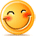:)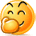:-)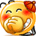:))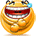=))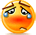:(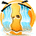:-(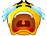:((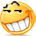:d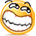:-d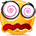@-)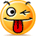:p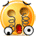:o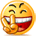:>)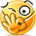(o)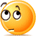[-(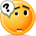:-?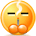(p)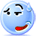:-s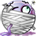(m)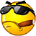8-)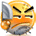:-t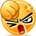:-b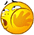b-(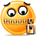:-#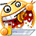=p~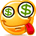:-\$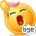(b)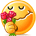(f)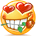x-)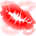(k)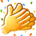(h)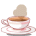(c)cheer